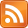Lucy and I found this proof last night. I'm sure it is not new, but hadn't seen it before.

We aim to prove that the square root of $3$ is irrational, i.e. that there is no rational $p$ such that $p^2 = 3$.

Proof: By contradiction. Suppose that there was such a $p$. Then, as a rational number, it could be written in lowest terms: i.e. as $\frac{m}{n}$ for $m, n \in \mathbb{Z}^+$ such that $m$ and $n$ are relatively prime.

$$\frac{m^2}{n^2} = 3$$

Consider the parities of $m$ and $n$. There are four options. Note that the parities of $m$ and $n$ determine the parities of $m^2$ and $n^2$.

$m$ even, $n$ even. This cannot happen since then $m$ and $n$ would share the factor $2$, violating our assumption that they were relatively prime.

$m$ even, $n$ odd. This cannot happen since an even number divided by an odd number is always even, but $3$ is odd.

$m$ odd, $n$ even. This cannot happen since an odd number divided by an even number is not an integer, but $3$ is an integer.

$m$ odd, $n$ odd. This possibility is the only one that is not ruled out immediately. But it too cannot happen. Write $m = 2j + 1$ and $n = 2k + 1$, where $j, k \in \mathbb{Z}^+$. Then:

$$\begin{matrix} \frac{(2j + 1)^2}{(2k + 1)^2} & = & 3 \\ (2j + 1)^2 & = & 3(2k + 1)^2 \\ 4j^2 + 4j + 1 & = & 3(4k^2 + 4k + 1) \\ \end{matrix}$$

Now consider the equation mod $4$. The left side is equal to $1$, but the right side is equal to $-1$, so we have a contradiction.

Notes: Consider using this proof to prove that the square root of $5$ is irrational. We also conclude that both $m$ and $n$ must be odd, but since $5 \equiv 1 \pmod{4}$ the last bit does not work. And indeed it better not, otherwise we could prove that the square root of $9$ was irrational.

This inelegant, bedraggled proof applies unevenly and will not win any beauty contests.